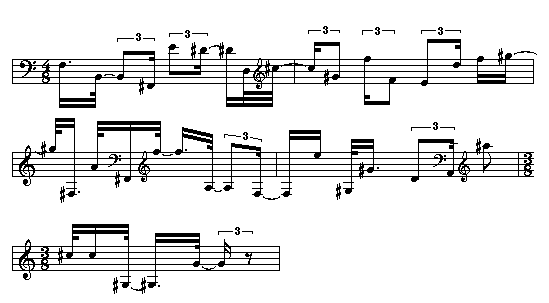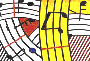Tutorial 27 - Quantification I

Introduction to rhythm quantification

Topics

Quantifying durations in millliseconds.

Functions used

omquantify,VOICE and flat.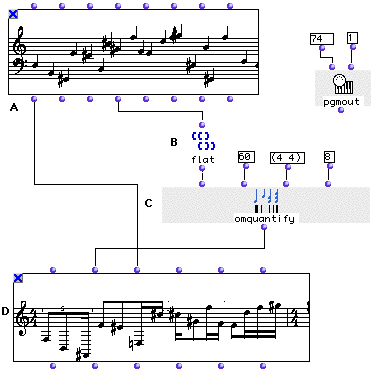Description

The omquantify function converts a list of milliseconds into a rhythmical tree (integers being durations and negative integers rests). In this example we will quantify a CHORD-SEQ (A) from tutorial 13 in two different fashion.

Patch structure

A: In order to quantify a sequence from a CHORD-SEQ we will use the list of durations that we get while evaluating the fourth output of CHORD-SEQ <ldur>. Each of these durations are related to each note in the CHORD-SEQ. In some cases we might have rests between notes. While using exclusively this method (using only the <ldur> output information of CHORD-SEQ) we will get a wrong quantification since <ldur> doesn't take in consideration rests since rests are not elements taken into account by the CHORD-SEQ object (see tutorial 23). Therefore we will open the CHORD-SEQ (A)editor and select the bottom left menu 'dur' in order to visualize durations. We will notice that in this sequence all notes fall one after another forming a monodic sequence without rests.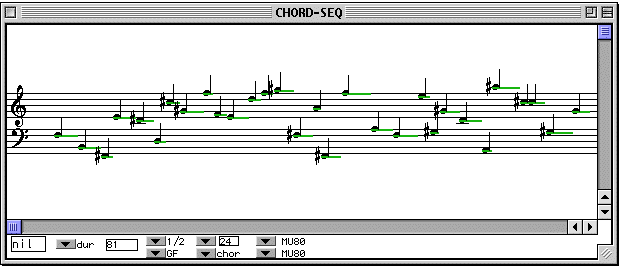B: We will then connect the fourth output <ldur>of the CHORD-SEQ (A) with a flat function (B) in order to get a simple list of duration

C: flat (B) is connected to the first input of omquantify (C) . In the second input we will enter 60 for the tempo. This will be the tempo in which durations will be quantified. The third input is for time signature. The fourth input is the maximum limit (exclusive) of rhytmical subdivisions. You may notice that these settings are equivalent to those found in the preferences when you choose the icon: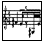then clicking on the qunatify icon: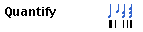the Quantify preferencespanel appears as: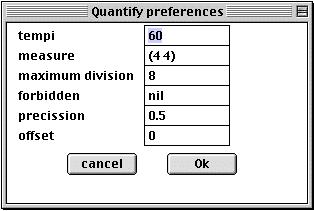These preferences are default values used for an internal method whenever we evaluate a VOICE factory connected to a CHORD-SEQ (the <self> output of a CHORD-SEQ is connected to the first input of a VOICE).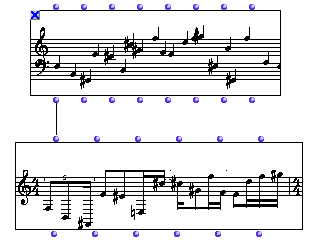D: We will connect the ouput of omquantify to the second input of VOICE since the output of omquantify is a tree. And in order to get the pitches as well, we will connect the <self> output of CHORD-SEQ to the third input <chords> of VOICE. Notice that we could also have used only the <lmidic> output of CHORD-SEQ since the VOICE factory can handle also a list of midicents instead of CHORD objects (see classes).

The rhythmical result will be: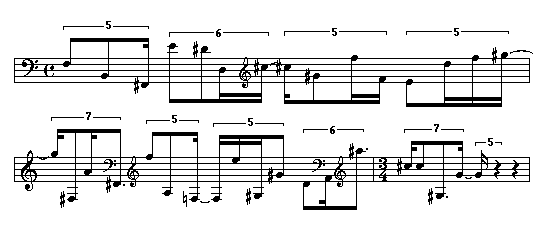E: Now let us simplify our rhythm and remove all irrationals by customizing our quantification values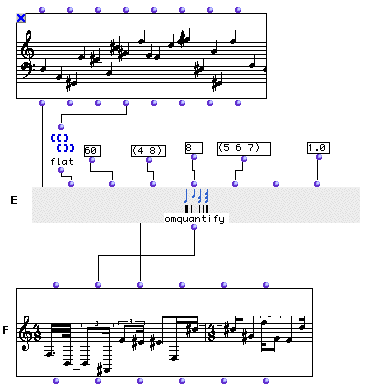In this case one must try many combinations between tempo and time signature settings. In the example above we have used the fifth and the seventh optionnal inputs of omquantify. In the fifth input is a list of forbidden rhythmical subdivisions (here quintuplets,sextuplets and seventuplets are forbidden). The seventh input is for precision. 1 is for the utmost precision, 0 is for the least. In our case, we had to change the time signature to (4 8), else the quantification skips the shortest notes and we ge a truncated rhythm. However this is always pointed out in the OM's Listener:

? Warning: with the given constraints, 1 notes are lost
while quantizing

The resulting rhythm is: